Dave's Math Tables: Conic Sections(Math | Algebra | Conics)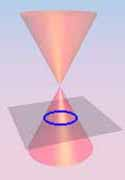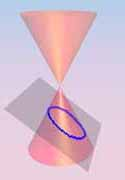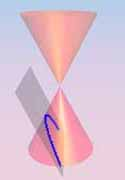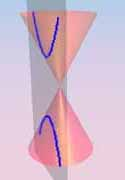Circle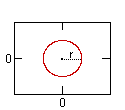Ellipse (h)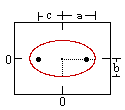Parabola (h)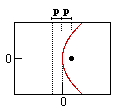Hyperbola (h)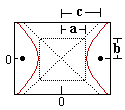Definition: A conic section is the intersection of a plane and a cone. Ellipse (v)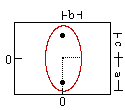Parabola (v)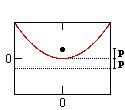Hyperbola (v)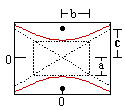By changing the angle and location of intersection, we can produce a circle, ellipse, parabola or hyperbola; or in the special case when the plane touches the vertex: a point, line or 2 intersecting lines.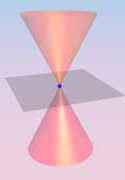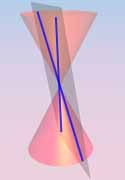Point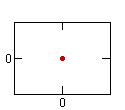LineDouble Line

 The General Equation for a Conic Section: Ax2 + Bxy + Cy2 + Dx + Ey + F = 0

The type of section can be found from the sign of: B2 - 4AC
 If B2 - 4AC is... then the curve is a... < 0 ellipse, circle, point or no curve. = 0 parabola, 2 parallel lines, 1 line or no curve. > 0 hyperbola or 2 intersecting lines.

The Conic Sections. For any of the below with a center (j, k) instead of (0, 0), replace each x term with (x-j) and each y term with (y-k).

 Circle Ellipse Parabola Hyperbola Equation (horiz. vertex): x2 + y2 = r2 x2 / a2 + y2 / b2 = 1 4px = y2 x2 / a2 - y2 / b2 = 1 Equations of Asymptotes: y = ± (b/a)x Equation (vert. vertex): x2 + y2 = r2 y2 / a2 + x2 / b2 = 1 4py = x2 y2 / a2 - x2 / b2 = 1 Equations of Asymptotes: x = ± (b/a)y Variables: r = circle radius a = major radius (= 1/2 length major axis) b = minor radius (= 1/2 length minor axis) c = distance center to focus p = distance from vertex to focus (or directrix) a = 1/2 length major axis b = 1/2 length minor axis c = distance center to focus Eccentricity: 0 c/a c/a Relation to Focus: p = 0 a2 - b2 = c2 p = p a2 + b2 = c2 Definition: is the locus of all points which meet the condition... distance to the origin is constant sum of distances to each focus is constant distance to focus = distance to directrix difference between distances to each foci is constant Related Topics: Geometry section on Circles